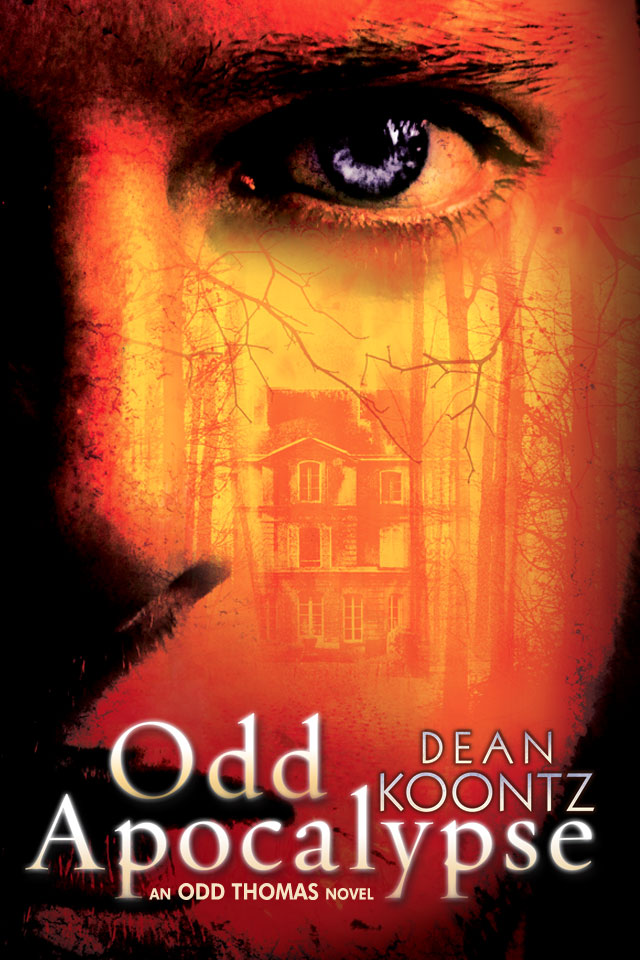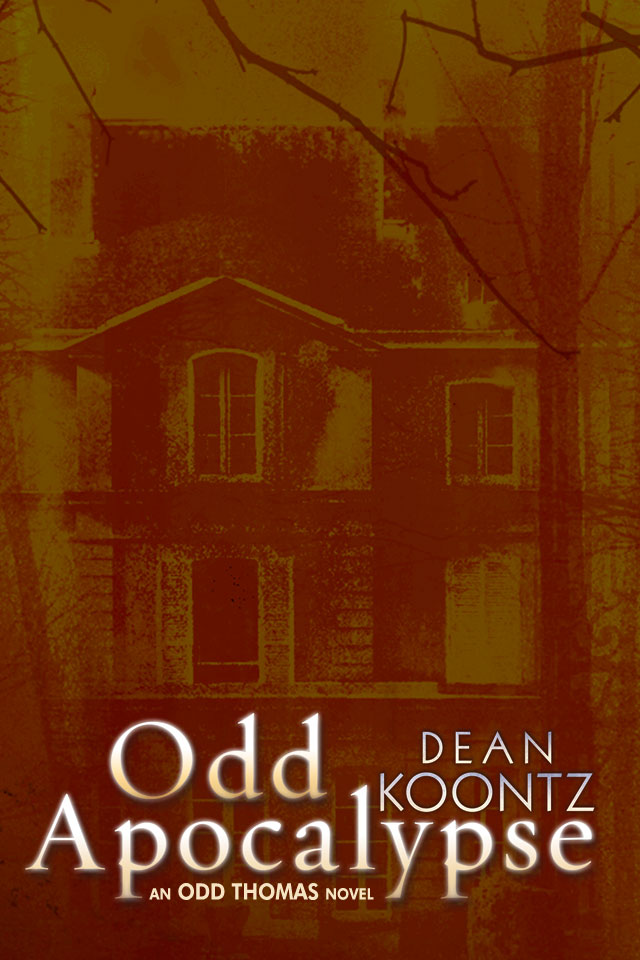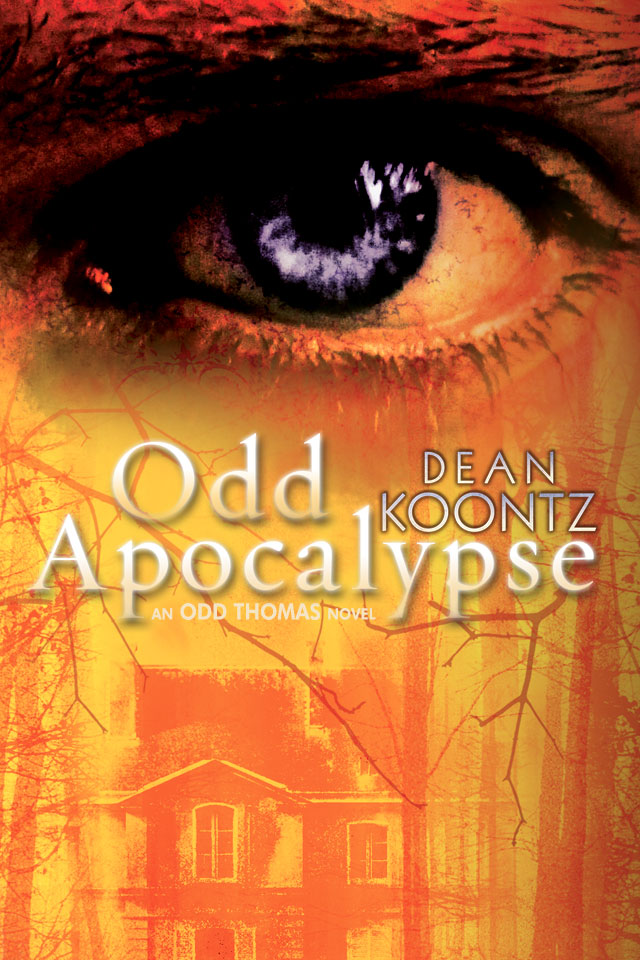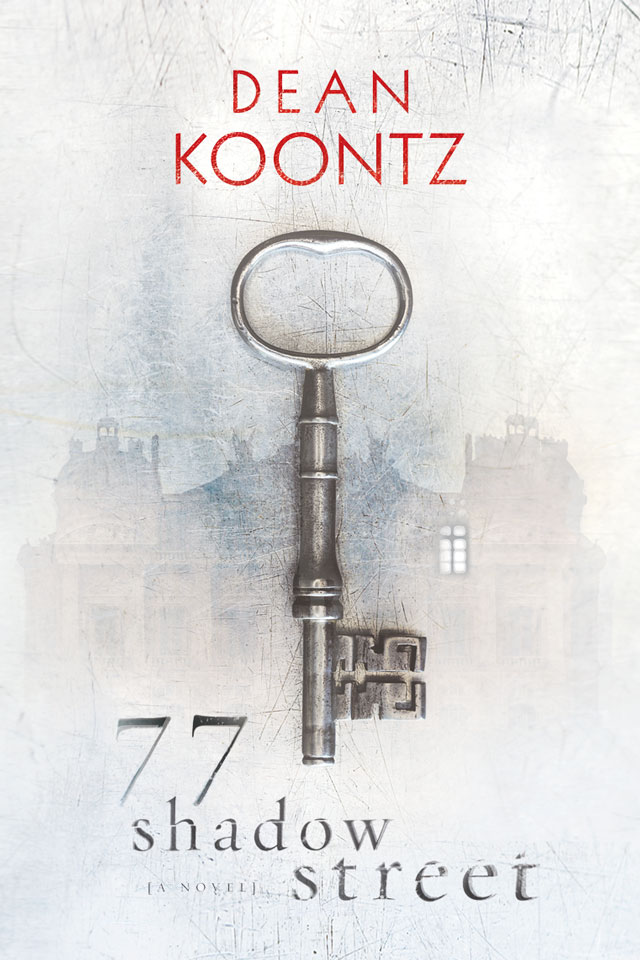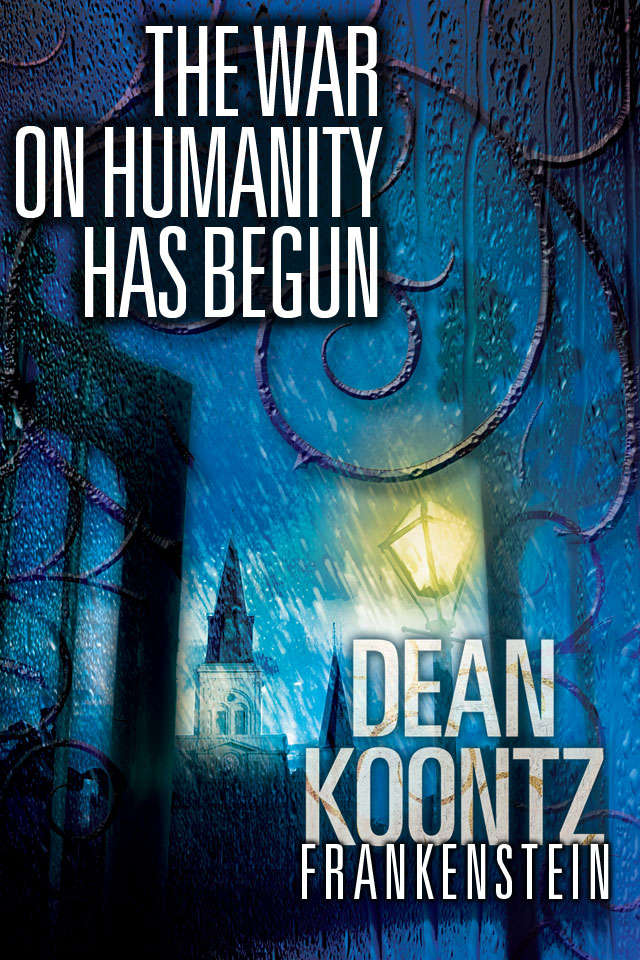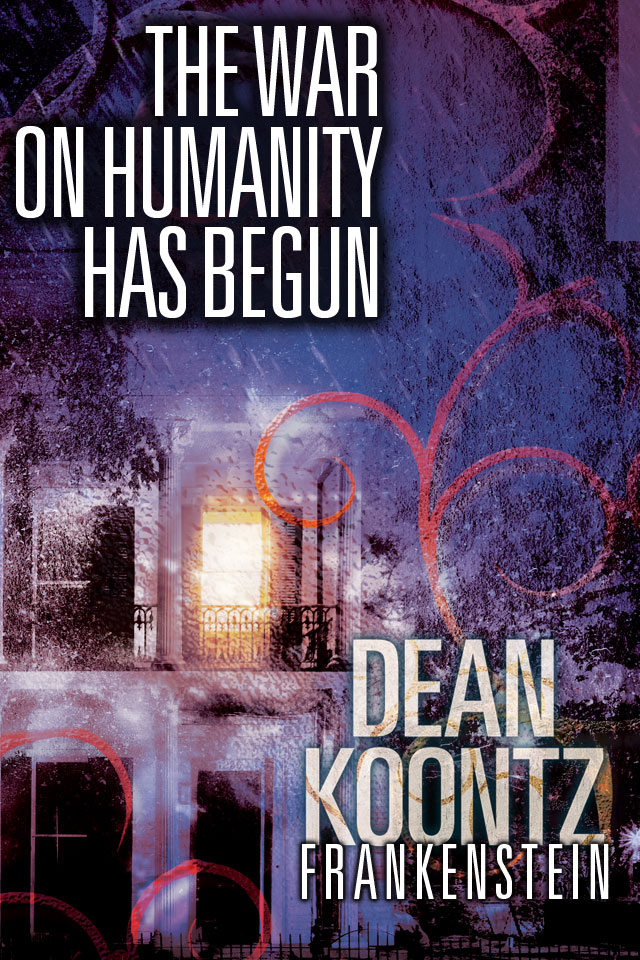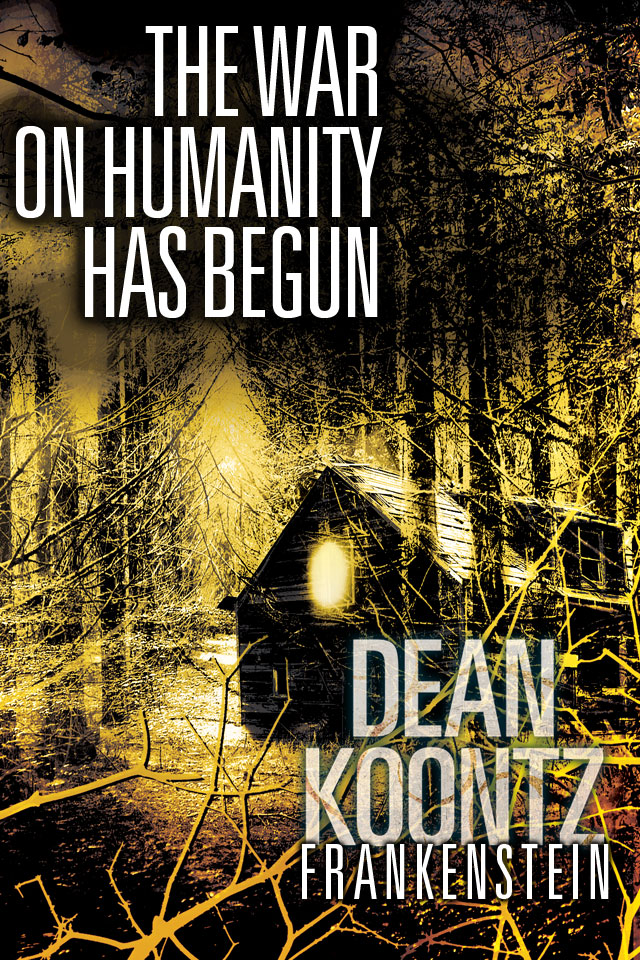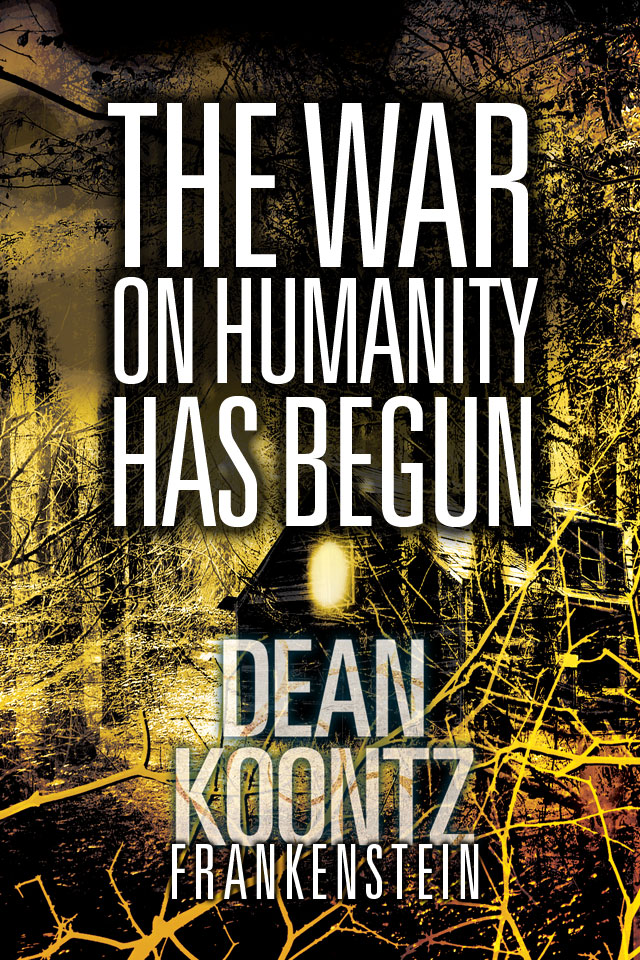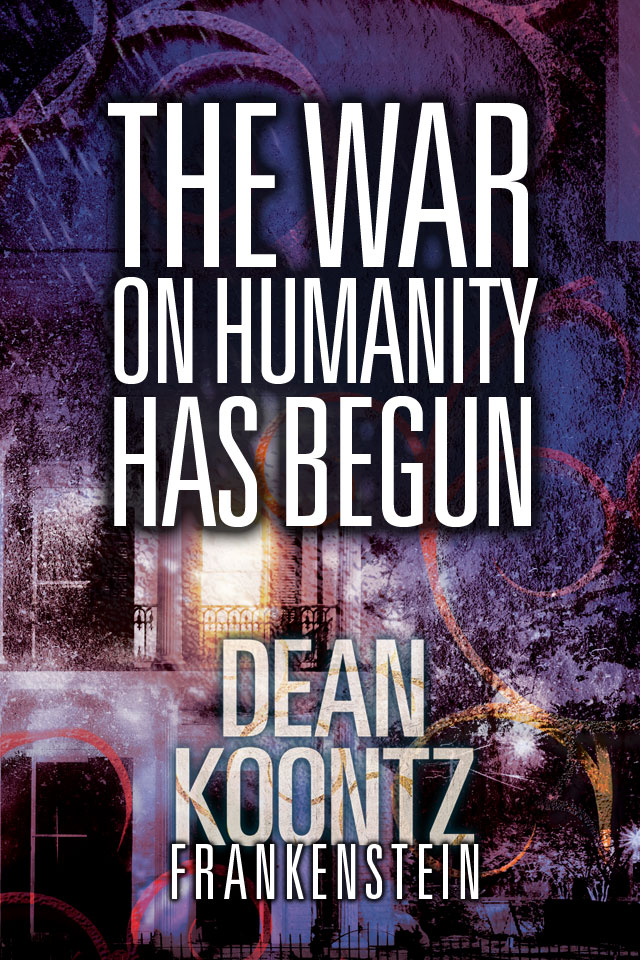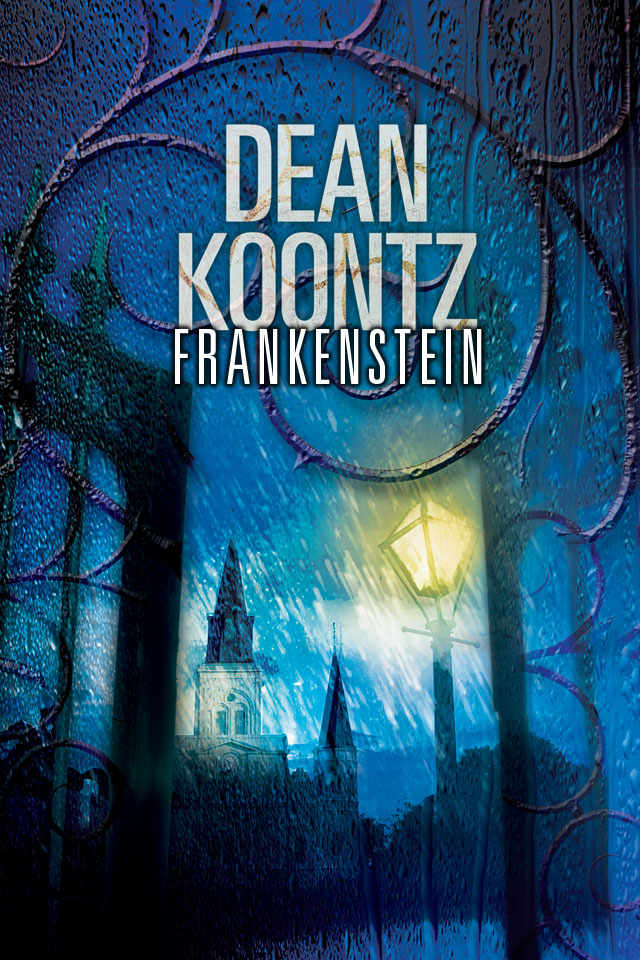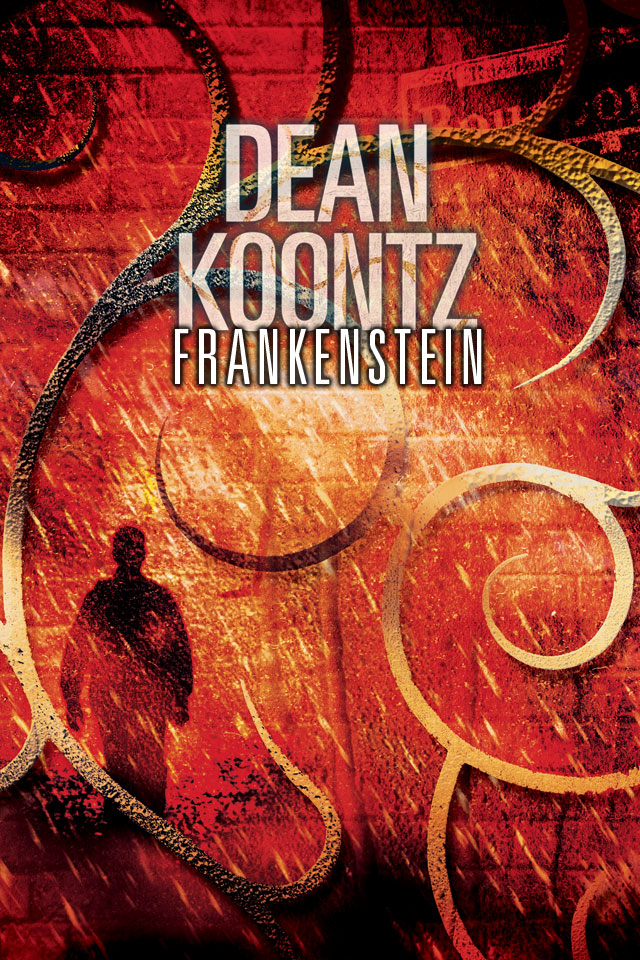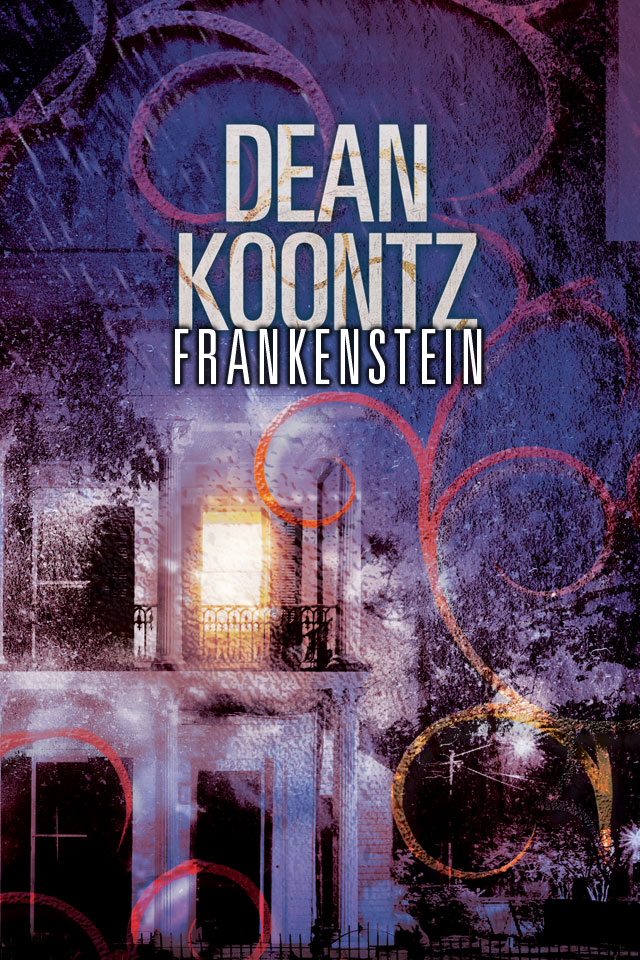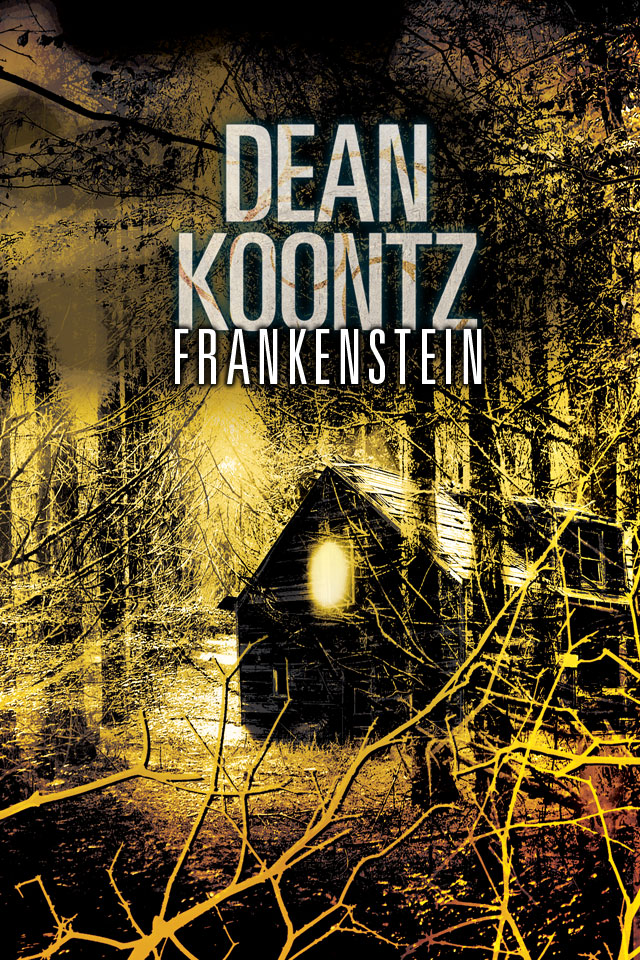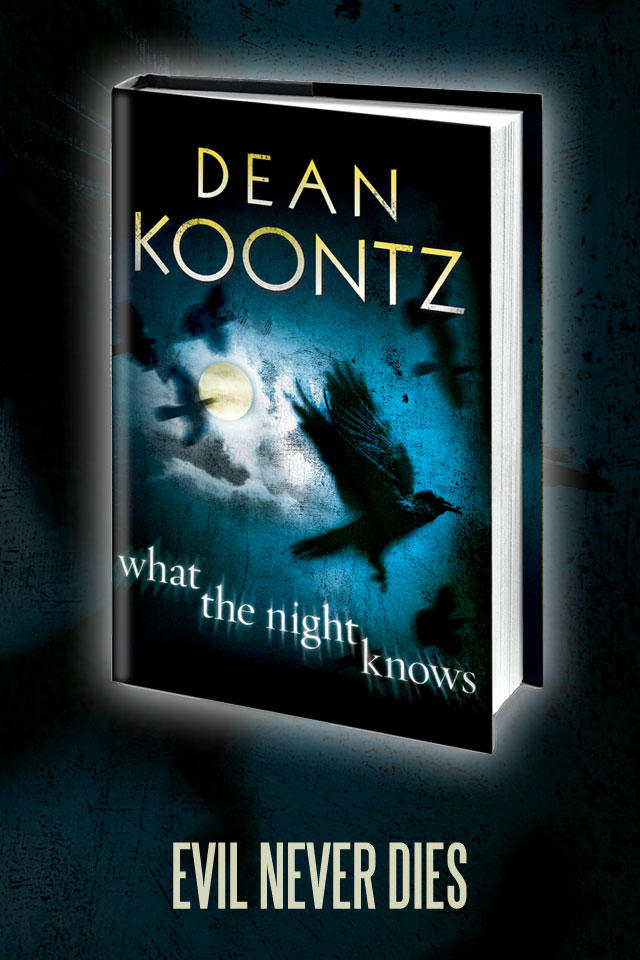# Wallpapers1024×768     1280×1024
1280×800     1440×900
1600×1024     1900×12801024×768     1280×1024
1280×800     1440×900
1600×1024     1900×12801024×768     1280×1024
1280×800     1440×900
1600×1024     1900×12801024×768
1280×800     1440×900
1600×1024     1920×10801024×768     1280×1024
1280×800     1440×900
1600×1024     1920×10801024×768     1280×1024
1280×800     1440×900
1600×1024     1920×10801024×768     1280×1024
1280×800     1440×900
1600×1024     1920×1080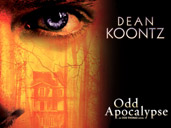1024×768     1280×1024
1280×800     1440×900
1600×1024     1920×1080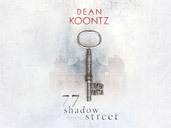1024×768     1280×1024
1280×800     1440×900
1600×1024     1920×12801024×768     1280×1024
1280×800     1440×900
1600×1024     1920×12801024×768     1280×1024
1280×800     1440×900
1600×1024     1920×12801024×768     1280×1024
1280×800     1440×900
1600×1024     1920×12801024×768     1280×1024
1280×800     1440×900
1600×1024     1920×12801024×768     1280×1024
1280×800     1440×900
1600×1024     1920×12801024×768     1280×1024
1280×800     1440×900
1600×1024     1920×12801024×768     1280×1024
1280×800     1440×900
1600×1024     1920×12801024×768     1280×1024
1280×800     1440×900
1600×1024     1920×12801024×768     1280×1024
1280×800     1440×900
1600×1024     1920×12801024×768     1280×1024
1280×800     1440×900
1600×1024     1920×12801024×768     1280×1024
1280×800     1440×900
1600×1024     1920×12801024×768     1280×1024
1280×800     1440×900
1600×1024     1920×12801024×768     1280×1024
1280×800     1440×900
1600×1024     1920×12801024×768     1280×1024
1280×800     1440×900
1600×1024     1920×12001024×768     1280×1024
1280×800     1440×900
1600×1024     1920×12001024×768     1280×1024
1280×800     1440×900
1600×1024     1920×12001024×768     1280×1024
1280×800     1440×900
1600×1024     1920×12001024×768     1280×1024
1280×800     1440×900
1600×1024     1920×12001024×768     1280×1024
1280×800     1440×900
1600×1024     1920×12001024×768     1280×1024
1280×800     1440×900
1600×1024     1920×1200

# Email Signatures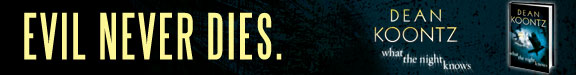# iPhone Wallpapers